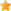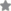### Design & Analysis of Computer AlgorithmsAuthor(s):
 Alfred V. Aho John E. Hopcroft Jeffrey D. Ullman
Author: Alfred V. Aho
• ISBN:9788131702055
• 10 Digit ISBN:8131702057
• Price:Rs. 899.00
• Pages:480
• Imprint:Pearson Education
• Binding:Paperback
• Status:Available

Ratings:The Design and Analysis of Computer Algorithms introduces the basic data structures and programming techniques often used in efficient algorithms. It covers the use of lists, push-down stacks, queues, trees, and graphs.

With this text, you gain an understanding of the fundamental concepts of algorithms, the very heart of computer science. It introduces the basic data structures and programming techniques often used in efficient algorithms. Covers use of lists, push-down stacks, queues, trees, and graphs. Later chapters go into sorting, searching and graphing algorithms, the string-matching algorithms, and the Schonhage-Strassen integer-multiplication algorithm. Provides numerous graded exercises at the end of each chapter.

### Table of Content

1. Models of Computation
2. Design of Efficient Algorithms
3. Sorting and Order Statistics.
4. Data Structures for Set Manipulation Problems.
5. Algorithms on Graphs.
6. Matrix Multiplication and Related Operations.
7. The Fast Fourier Transform and its Applications.
8. Integer and Polynomial Arithmetic.
9. Pattern-Matching Algorithms.
10. NP-Complete Problems.
11. Some Provably Intractable Problems.
12. Lower Bound on Numbers of Arithmetic Operations